x-1 (number)Encyclopedia

In mathematics
Mathematics
Mathematics is the study of quantity, space, structure, and change. Mathematicians seek out patterns and formulate new conjectures. Mathematicians resolve the truth or falsity of conjectures by mathematical proofs, which are arguments sufficient to convince other mathematicians of their validity...

, −1 is the additive inverse
In mathematics, the additive inverse, or opposite, of a number a is the number that, when added to a, yields zero.The additive inverse of a is denoted −a....

of 1, that is, the number that when added
Addition is a mathematical operation that represents combining collections of objects together into a larger collection. It is signified by the plus sign . For example, in the picture on the right, there are 3 + 2 apples—meaning three apples and two other apples—which is the same as five apples....

to 1 gives the additive identity element, 0. It is the negative integer
Integer
The integers are formed by the natural numbers together with the negatives of the non-zero natural numbers .They are known as Positive and Negative Integers respectively...

greater than negative two (−2) and less than 0
0 (number)
0 is both a numberand the numerical digit used to represent that number in numerals.It fulfills a central role in mathematics as the additive identity of the integers, real numbers, and many other algebraic structures. As a digit, 0 is used as a placeholder in place value systems...

.

Negative one has some similar but slightly different properties to positive one.

Negative one bears relation to Euler's identity since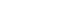In computer science
Computer science
Computer science or computing science is the study of the theoretical foundations of information and computation and of practical techniques for their implementation and application in computer systems...

, −1 is a common initial value for integers and is also used to show that a variable contains no useful information.

## Algebraic properties

Multiplying a number by −1 is equivalent to changing the sign on the number. This can be proved using the distributive law and the axiom that 1 is the multiplicative identity: for x real
Real number
In mathematics, a real number is a value that represents a quantity along a continuum, such as -5 , 4/3 , 8.6 , √2 and π...

, we have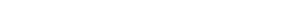where we used the fact that any real x times 0 equals 0, implied by cancellation
Cancellation property
In mathematics, the notion of cancellative is a generalization of the notion of invertible.An element a in a magma has the left cancellation property if for all b and c in M, a * b = a * c always implies b = c.An element a in a magma has the right cancellation...

from the equation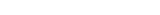In other words,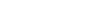so (−1) · x is the arithmetic inverse of x, or −x.

### Square of −1

The square of −1, i.e. −1 multiplied by −1, equals 1. As a consequence, a product of negative real numbers is positive.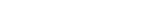The first equality follows from the above result. The second follows from the definition of −1 as additive inverse of 1: it is precisely that number that when added to 1 gives 0. Now, using the distributive law, we see that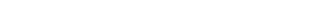The second equality follows from the fact that 1 is a multiplicative identity. But now adding 1 to both sides of this last equation impliesThe above arguments hold in any ring
Ring (mathematics)
In mathematics, a ring is an algebraic structure consisting of a set together with two binary operations usually called addition and multiplication, where the set is an abelian group under addition and a semigroup under multiplication such that multiplication distributes over addition...

, a concept of abstract algebra
Abstract algebra
Abstract algebra is the subject area of mathematics that studies algebraic structures, such as groups, rings, fields, modules, vector spaces, and algebras...

generalizing integers and real numbers.

### Square roots of −1

The complex number
Complex number
A complex number is a number consisting of a real part and an imaginary part. Complex numbers extend the idea of the one-dimensional number line to the two-dimensional complex plane by using the number line for the real part and adding a vertical axis to plot the imaginary part...

i
Imaginary unit
In mathematics, the imaginary unit allows the real number system ℝ to be extended to the complex number system ℂ, which in turn provides at least one root for every polynomial . The imaginary unit is denoted by , , or the Greek...

satisfies i2 = −1, and as such can be considered as a square root
Square root
In mathematics, a square root of a number x is a number r such that r2 = x, or, in other words, a number r whose square is x...

of −1. The only other complex number x satisfying the equation x2 = −1 is −i. In the algebra of quaternion
Quaternion
In mathematics, the quaternions are a number system that extends the complex numbers. They were first described by Irish mathematician Sir William Rowan Hamilton in 1843 and applied to mechanics in three-dimensional space...

s, containing the complex plane, the equation x2 = −1 has an infinity of solutions.

## Exponentiation to negative integers

Exponentiation
Exponentiation
Exponentiation is a mathematical operation, written as an, involving two numbers, the base a and the exponent n...

of a non-zero real number can be extended to negative integers. We make the definition that x−1 = 1/x, meaning that we define raising a number to the power −1 to have the same effect as taking its reciprocal. This definition then extended to negative integers preserves the exponential law xaxb = x(a + b) for a,b non-zero real numbers.

Exponentiation to negative integers can be extended to invertible elements of a ring, by defining x−1 as the multiplicative inverse of x.

## Computer representation

There are a variety of ways that −1 (and negative numbers in general) can be represented in computer systems, the most common being as two's complement
Two's complement
The two's complement of a binary number is defined as the value obtained by subtracting the number from a large power of two...

of their positive form. Since this representation could also represent a positive integer in standard binary representation, a programmer must be careful not to confuse the two. Negative one in two's complement could be mistaken for the positive integer 2n − 1, where n is the number of digits in the representation (that is, the number of bits in the data type). For example, 111111112 (binary) and FF16 (hex) each represents −1 in two's complement, but represents 255 in standard numeric representation.